Time: 3 Hours                                                                                                     Max. Marks: 100

NOTE: There are 9 Questions in all.

·      Question 1 is compulsory and carries 20 marks. Answer to Q. 1. must be written in the space provided for it in the answer book supplied and nowhere else.

·      Out of the remaining EIGHT Questions answer any FIVE Questions. Each question carries 16 marks.

·      Any required data not explicitly given, may be suitably assumed and stated.

Q.1       Choose the correct or best alternative in the following:                       (2x10)

a.  The average number of key comparisons done in successful sequential search in a list of length n is

(A)  log n                                              (B)  (n-1)/2

(C)  n/2                                                (D)  (n+1)/2

b.      The maximum number of nodes in a binary tree of depth 5 is

(A)  31                                                (B)  16

(C)  32                                                (D)  15

c.   n elements of a Queue are to be reversed using another queue. The number of “ADD” and “REMOVE” operations required to do so is

(A)  2*n                                              (B)  4*n

(C)  n                                                  (D)  The task cannot be accomplished

d.   A complete binary tree with n leaf nodes has

(A)  n+1 nodes                                    (B)  2n-1 nodes

(C)  2n+1 nodes                                  (D)  n(n-1)/2 nodes

e.   A binary tree can be converted in to its mirror image by traversing it in

(A)     Inorder                                        (B)  Preorder

(C)  Postorder                                     (D)  Anyorder

f.    One can convert an infix expression to a postfix expression using a

(A)  stack                                            (B)  Queue

(C)  Deque                                          (D)  none of these

g.   Which of the following types of expressions do not require precedence rules for evaluation?

(A)   fully parenthesised infix expression

(B)   postfix expression

(C)   partially parenthesised infix expression

(D)  more than one of the above

h.   Overflow condition in linked list may occur when attempting to_____

(A)    Create a node  when free space pool is empty.

(B)    Traverse the nodes when free space pool is empty.

(C)    Create a node when linked list is empty.

(D)    None of these.

i.    Linked lists are not suitable data structures for which one of the following problems

(A)  insertion sort                                 (B)  binary search

(C)  radix sort                                      (D)  polynomial manipulation

j.    The sorting technique where array to be sorted is partitioned again and again in such a way that all elements less than or equal to partitioning element appear before it and those which are greater appear after it, is called

(A)  merge sort                                    (B)  quick sort

(C)  selection sort                                (D)  none of these

Answer any FIVE Questions out of EIGHT Questions.

Each question carries 16 marks.

Q.2     a.   Sort the following list using selection Sort. Show each pass of the sort.

45, 25, 75, 15, 65, 55, 95, 35                                                                           (7)

b.      What is Hashing? Can a perfect Hash function be made? Justify your answer. Explain in brief the various methods used to resolve collision.         (9)

Q.3     a.   Define a B tree of order m.

Write algorithms to

(i)                  Search for a key in B-tree.

(ii)                Insert a key in a B-tree.

(iii)               Delete a key from a B-tree.                                             (10)

b.   Given the following tree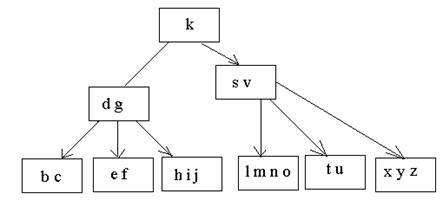Show how the tree will change when the following steps are executed one after another

(i)                  Key ‘i’ is deleted

(ii)                Key ‘v’ is deleted

(iii)               Key ‘t’ is deleted                                                                       (6)

Q.4     a.   Write an algorithm to reverse a singly linked list.                                                 (4)

b.        Two stacks are implemented using an array. What should be the initial values of top for the two stacks? Arrows show the direction of growth of the stack.

Array A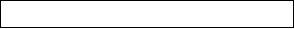S1                                                                            S2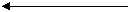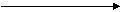How can we implement m stacks in a contiguous a memory. Explain how the stacks will grow and indicate the boundary condition. Write push and pop programs for such a scenario.                         (10)

c.   Consider a 2D array as char A  stored in contiguous memory locations starting from location 1000” in column major order. What would the address of a[i] [d]?                                                   (2)

Q.5     a.   What are priority Queues? How can priority queues be implemented? Explain in brief.                    (4)

b.   Consider  a linked list with n integers. Each node of the list is numbered from ‘1’ to ‘n’. Write an algorithm to split  this list into 4 lists so that

first list contains nodes numbered 1,5, 9, 13- - -

second list contains nodes numbered 2, 6, 10, 14- - -

third list contains nodes numbered 3, 7, 11, 15- - -

and fourth list contains nodes numbered 4,8, 12, 16- - -                                     (8)

c.       Write a recursive algorithm to find Greatest Common Divisor of two integers  with the help of Euclid’s algorithm, as per the following formula(4)

Q.6     a.   Traverse the following binary tree in inorder,  preorder and postorder.                (3)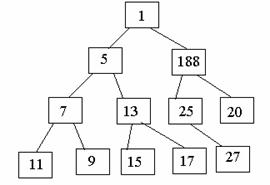b.   Define the following:

(i)                  Tree ( recursive defination)

(ii)                Level of a node.

(iii)               Height of a tree.

(iv)              Complete Binary  tree.

(v)                Internal Path length.                                                        (2+1+1+2+1)

c.   What is an AVL tree? Explain how a node can be inserted into an AVL tree.     (6)

Q.7     a.   Describe Kruskal’s algorithm to find minimum spanning trees.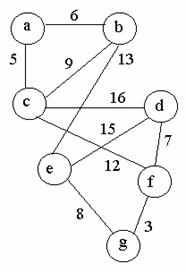Apply Kruskal’s algorithm for the above graph.                                               (10)

b.   Differentiate between (i) Top-down and Bottom-up programming?

(ii) Structured and Modular programming.                                                          (6)

Q.8     a.   How is recursion handled internally. Explain with the help of a suitable example.                    (4)

b.   Write a an algorithm to add two polynomials using linked list.                   (12)

Q.9           Execute Dijkstra’s algorithm on the following graph with vertices numbered 1 to 6. The graph is given in the form of an adjacency list.

1        : (6, 2), (4, 7)

2        : (1,3), (3,4)

3        : (5,6)

4        : (2,2) (3,5), (1,5), (5,1)

5        : (4,4), (6,3)

6        : (4,3)

Here (3,4) in vertex 2’s adjacency list means that vertex 2 has a directed edge to vertex 3 with a weight of 4. Assuming the source vertex to be 2, find the shortest distance and the path to all the remaining vertices.                                                           (16)